# Application of Python Matplotlib color

2022-02-01 13:20:57This is my participation 11 The fourth of the yuegengwen challenge 26 God , Check out the activity details ：2021 One last more challenge

# Preface

matplotlib Modules are very powerful ,pyplot Class provides users with the ability to quickly draw polylines 、 Columnar 、 Script methods for common charts such as scatter points . meanwhile ,matplotlib Rely on many underlying renderers, such as Agg Display of image data processing .

In order to draw more beautiful images , We all use matplotlib Apply colors in the image .

In the previous study , There are two main ways to apply colors in charts ：

• Set the color fill attribute keyword ： Such as plot、bar、hist、pi、contour And so on facecolor/color/cmap Attribute keyword
• Rendering numpy data ： Use imshow()/pcolor Method display numpy The data is rendered into an image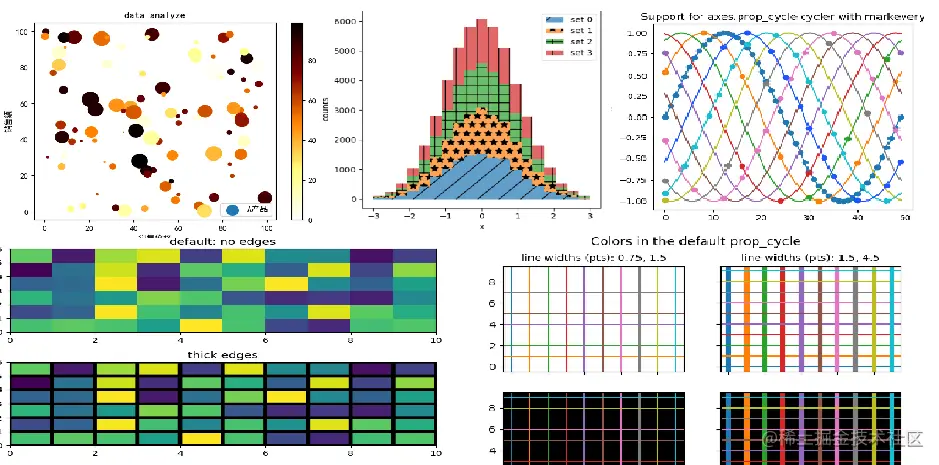In this issue , We will be on matplotlib Draw a color table in the module 、 Chart colors should be learned by applying relevant method attributes ,let's go~

# 1. imshow() Draw a color table

• ## imshow() Methods an overview

pyplot.imshow() Yes, it will numpy The generated data is rendered into 2D Images

• imshow() take RGBA Data or 2D Rendering scalar data into a color image
• imshow() Can pass cmap|vmin|vmax Specifies the color level of the output
• ## imshow() Method to draw a color table

• Import matplotlib.pyplot library
• call numpy.random.randint() Generate vector data
• call pyplot.imshow() Render the data into an image
• call pylot.show() Show the image
``````x = np.random.randint(1,100,size=(3,5))
plt.imshow(x)
plt.show()
Copy code ``````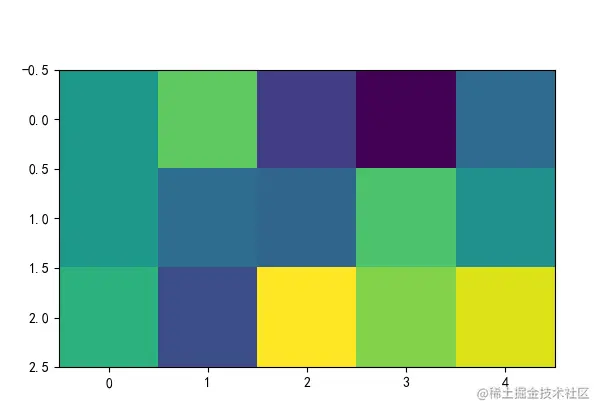• Can pass cmap、vmin、vmax Change the render color level

`````` plt.imshow(x,cmap="hot",vmin=10,vmax=210)
Copy code ``````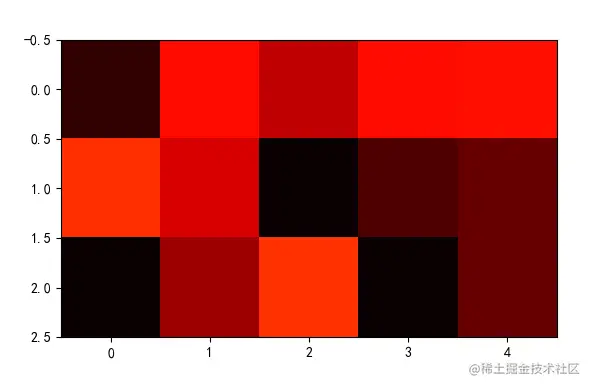• You can call text() Method to fill each color text

``````fig,ax = plt.subplots()

x = np.random.randint(1,100,size=(7,7))

ax.imshow(x,cmap="magma_r")

for i in range(7):
for j in range(7):
text = ax.text(j,i,x[i,j],ha="center",va="center",color="w")

plt.show()
Copy code ``````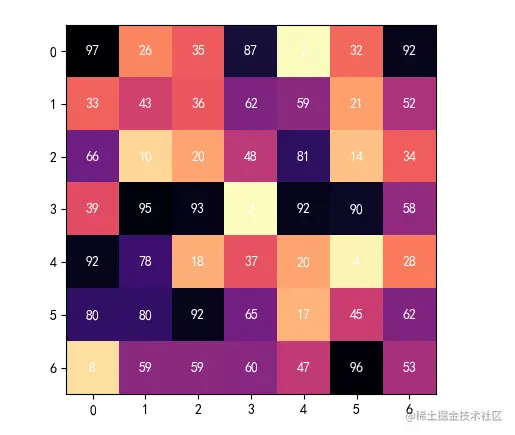# 2. pcolormesh() Draw a color table

• ## pcolor() Methods an overview

• pcolor() Method creates an unconventional color mesh using quadrilateral
• pcolor() Method for large matrices , Rendering will be slow
• pcolor（） Method only supports for x,y Mask for processing
• ## pcolormesh() Methods an overview

• pcolormesh() Method creates a colored grid using a square
• pcolormesh() The method is suitable for large matrix data
• pcolormesh() Method will mask the of the element facecolor Set transparent , You can see the difference using the edge color
• ## pcolor() Method practice

``````x = np.random.rand(6,10)

plt.pcolor(x)

plt.colorbar()

plt.show()
Copy code ``````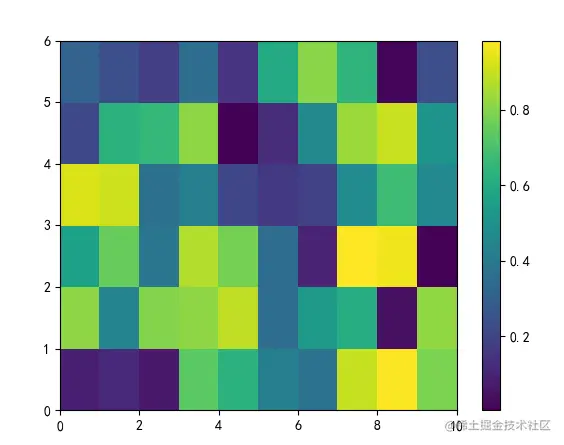• ## pcolormesh() Method practice

``````x = np.random.rand(6,10)

plt.pcolormesh(x,edgecolors="k")

plt.colorbar()

plt.show()
Copy code ``````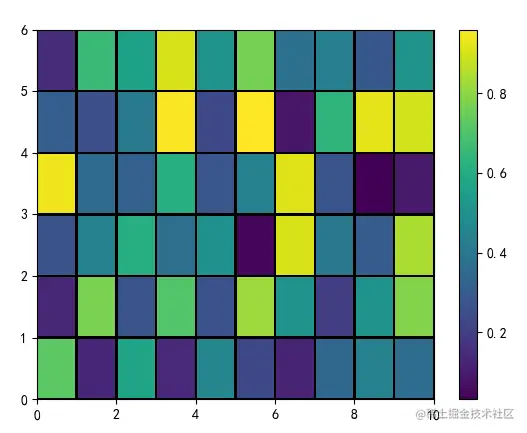# 3. hline()、vline() Draw colored lines

• ## hline()、vline() Methods an overview

• pyplot.hline(y,xmin,xmax) Method to draw a horizontal line
• pyplot.vline(x,ymin,ymax) Method to draw a vertical line
• ## hline()、vline() Method practice

• call pyplot.rcParams['axes.prop_cycle'].by_key()['color'] obtain Axes The color of the object
• call pyplot.vline()、pyplot.hline() Method to draw a vertical horizontal line
``````prop_cycle = plt.rcParams['axes.prop_cycle']
colors = prop_cycle.by_key()['color']

for i,color in enumerate(colors):

plt.vlines(i,0,10,color=color)
plt.hlines(i,0,10,color=color)

plt.show()
Copy code ``````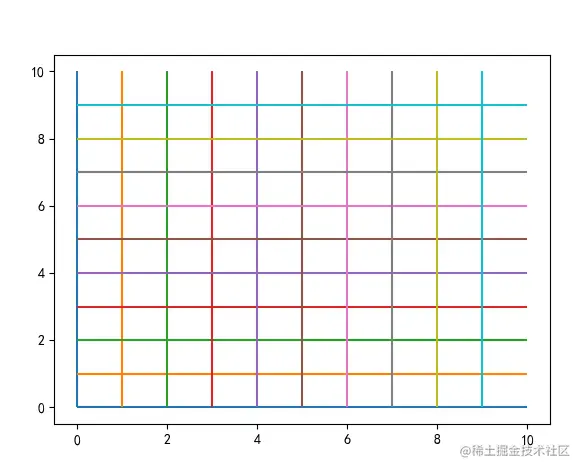# 4. colorbar Draw color bars

• ## colorbar() Methods an overview

• pylot.colorbar Add a color bar to the chart
• colorbar It can be applied to scatter、contour、imshow、pcolormesh in
• colorbar In the chart, the default is vertical display , Can pass orientation Set level
• ## colorbar() Method practice

``````data = np.arange(100).reshape(10,10)

im = plt.imshow(data)

plt.colorbar(im,orientation="horizontal")

plt.show()
Copy code ``````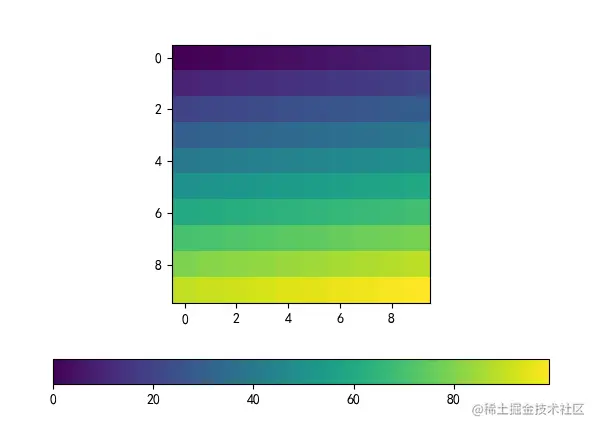# 5. Chart color properties

• ## Color attribute keyword

• cmap:RGBA Color mapping table , In the form of " Color map table name _r"
• color: RGBA Color tuples or lists
• facecolor: Graphic color
• edgecolor: Graphic border color
• Color value form ：
• English words for color ： Like red "red"
• Abbreviations of words indicating color, such as ： Red "r", yellow "y"
• RGB Format ： Hexadecimal format, such as "#88c999";(r,g,b) Tuple form
• You can go to the color list
• ## List of common chart color attributes

Method Chart name cmap color facecolor edgecolor
pyplot.hist() Histogram × ×
pyplot.plot() Broken line diagram × × ×
pyplot.bar() Histogram ×
pyplot.pie() The pie chart × √(colors) ×
pyplot.scatter() Scatter plot √ (c) × √(edgecolors)
pyplot.contour() Contour map √(colors) × ×
pyplot.boxplot() Box figure × × × ×
pyplot.violinplot() Picture of violin × × × ×
pyplot.imshow() Exhibition dta For image × × ×
pyplot.pcolor() Color grid √(edgecolors)

# summary

In this issue , We are right. matplotlib When charting in the module , The application methods and properties of color are summarized . How to show the data clearly , Colors are often used in charts to help us distinguish .

The above is the content of this issue , Welcome big guys to praise and comment , See you next time ~